# Elastic Collisions

## Elastic Collisions

• Elastic collision is a collision where the both kinetic energy and Linear Momentum is conserved
• Coefficient of restitution for the Elastic collision is 1
• Elastic collision can be further divided into head on collision (i.e collision in one dimension) and opaque collision (i.e collision in two dimension)
• If the initial velocities and final velocities of both the bodies are along the same straight line, then it is called a one-dimensional collision, or head-on collision. In the case of small spherical bodies, this is possible if the direction of travel of body 1 passes through the centre of body 2 which is at rest.
• the collision is two dimensional, where the initial velocities and the final velocities lie in a plane
• Lets study about these two elastic collision in one dimension and two dimension in further details

## Head on elastic collision of two particles

• Consider two particles whose masses are m1 and m2 respectively and they collide each other with velocity u1 and u2 and after collision their velocities become v1 and v2 respectively.
• Collision between these two particles is head on elastic collision. From law of conservation of momentum we have
m1u1 + m2u2 = m1v1 + m2v2                      (1)
and from law of conservation of kinetic energy for elastic collision we have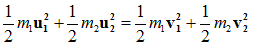(2)
rearranging equation 1 and 2 we get
m1(u1-v1)= m2(v2-u2)                     (3)
and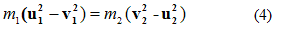dividing equation 4 by 3 we get
u1 + v1 = u2 + v2
u2 - u1 = -(v2 - v1)                     (5)
where (u2 - u1) is the Relative Velocity of second particle w.r.t. first particle before collision and (v2 - v1) is the relative velocity of second particle w.r.t. first after collision.
• From equation 5 we come to know taht in a perfectly elastic collision the magnitude of relative velocity remain unchanged but its direction is reversed. With the help of above equations we can find the values of v2 and v1 , so from equation 5
v1 = v2 - u1 + u2                     (6)
v2 = v1 + u1 - u2                     (7)
Now putting the value of v1 from equation 6 in equation 3 we get
m1(u1 - v2 + u1 - u2) = m2(v2 - u2)
On solving the above equation we get value of v2 as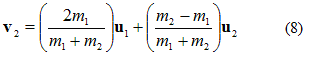• Similarly putting the value of v2 from equation 7 in equation 3 we get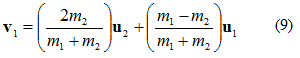• Total kinetic energy of particles before collision is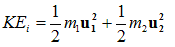and total K.E. of particles after collision is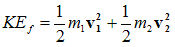• Ratio of initial and final K.E. is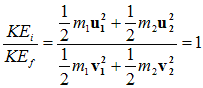• Special cases
Case I: When the mass of both the particles are equal i.e., m1 = m2 then from equation 8 and 9 , v2=u1 and v1=u2. Thus if two bodies of equal masses suffer head on elastic collision then the particles will exchange their velocities. Exchange of momentum between two particles suffering head on elastic collision is maximum when mass of both the particles is same.

Case II: when the target particle is at rest i,e u2=0
From equation (8) and (9)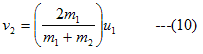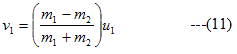Hence some part of the KE which is transformed into second particle would be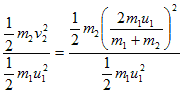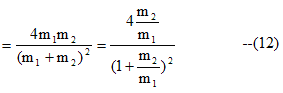when m1=m2,then in this condition v0=0 and v2=u1 and part of the KE transferred would be
=1

Therefore after collisom first particle moving with initial velocity u1 would come to rest and the second particle which was at rest would start moving with the velocity of first particle.Hence in this case when m1=m2 transfer of energy is 100%.if m1 > m2 or
m1 < m2 ,then energy transformation is not 100%

Case III:
if m2 >>>> m1 and u2=0 then from equation (10) and (11)
v1 ≅ -u1 and v2=0                     (13)
For example when a ball thrown upwards collide with earth

Case IV:
if m1 >>>> m2 and u2=0 then from equation (10) and (11)
v1 ≅ u1 and v2=2u1                     (14)

Therefore when a heavy particle collide with a very light particle at rest ,then the heavy particle keeps on moving with the same velocity and the light particle come in motion with a velocity double that of heavy particle

## (15) Deflection of an moving particle by a particle at rest during perfectly elastic collision in two dimension

• Let m1 and m2 be the two mass particle in a laboratory frame of refrence and m1 collide with m2 which is initailly is at rest.Let the velocity of mass m1 before collison be u1 and after the collison it moves with a velocity v1 and is delfected by the angle θ1 withs its incident direction and
m2 after the collision moves with the velocity v2 and it is deflected by an angle θ2 with its incident direction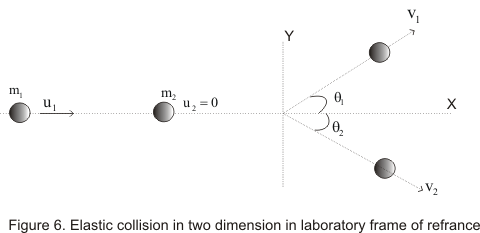• From law of conservation of linear momentum ,for components along x-axis
m1u1=m1v1cosθ1 +m2v2cosθ2 ---(1)
For components along y-axis
0=m1v1sinθ1 -m2v2sinθ2 --(2)
And from law of conservation of energy
(1/2)m1u12=(1/2)m1v12+(1/2)m2v22 ---(3)

• Analysing above equations we come to know that we have to find values of four unknown quantities v1,v212 with the help of above three equations which is not possible. So we cannot predict the variable as they are four of them . However if we measure any one variable ,then other variable can be uniquely determined from the above equation